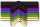# Search Results

Searching for: if

## Individual Exercises

0 / 50

Write a function in Java that implements the following logic: Given 2 ints, a and b, return their sum. However, sums in the range 10..19...0 / 50

Write a function in Java that implements the following logic: Given three ints, a b c, return true if one of them is 10 or more less than...0 / 50

Write a function in Java that implements the following logic: Given a number n, return true if n is in the range 1..10, inclusive. Unless...0 / 50

Write a function in Java that implements the following logic: We are having a party with amounts of tea and candy. Return the int outcome...0 / 50

Write a function in Java that implements the following logic: Your cell phone rings. Return true if you should answer it. Normally you...0 / 50

Write a function in Java that implements the following logic: Given a string, return true if it ends in "ly".0 / 50

Write a function in Java that implements the following logic: Given a string, if the string begins with "red" or "blue" return that color...0 / 50

Write a function in Java that implements the following logic: The web is built with HTML strings like "<i>Yay</i>" which...0 / 50

Write a function in Java that implements the following logic: Given 2 strings, a and b, return a string of the form short+long+short,...0 / 50

Write a function in Java that implements the following logic: Given 2 ints, `a` and `b`, return their sum. However, "teen" values in the...0 / 50

Write a function in Java that implements the following logic: The squirrels in Palo Alto spend most of the day playing. In particular,...0 / 50

Write a function in Java that implements the following logic: You are driving a little too fast, and a police officer stops you. Write...0 / 50

Write a function in Java that implements the following logic: Given a non-negative number `num`, return true if `num` is within 2 of a...0 / 50

Write a function in Java that implements the following logic: Given three ints, `a`, `b`, and `c`, return true if `b` is greater than `a`, and `c` is...0 / 50

Write a function in Java that implements the following logic: Given three ints, `a`, `b`, and `c`, return true if it is possible to add two of...0 / 50

Write a function in Java that implements the following logic: You have a red lottery ticket showing ints `a`, `b`, and `c`, each of which is 0,...0 / 50

Write a function in Java that implements the following logic: You have a blue lottery ticket, with ints `a`, `b`, and `c` on it. This makes...0 / 50

Write a function in Java to implement the following logic: We want to make a row of bricks that is `goal` inches long. We have a number of...0 / 150

Write a function in Java that implements the following logic: Given 3 int values, `a`, `b`, and `c`, return their sum. However, if one of the...0 / 50

Write a function in Java that implements the following logic: Given 3 int values, `a`, `b`, and `c`, return their sum. However, if one of the...0 / 50

Write two methods in Java that implements the following logic: Given 3 int values, `a`, `b`, and `c`, return their sum. However, if any of the...0 / 50

Write a function in Java that implements the following logic: Given 2 `int` values greater than 0, return whichever value is nearest to 21...0 / 50

Write a function in Java that implements the following logic: Given three ints, `a`, `b`, and `c`, one of them is small, one is medium and one...0 / 50

Write a function in Java that implements the following logic: Given a string, return a string made of its first 2 chars. If the string...0 / 50

Write a function in Java that implements the following logic: Given a string, return a string of length 1 from its front, unless `front` is...